# Abstract

We reconsider a nonlinear quantum kinetic theory which is built within the context of a nonequilibrium statistical ensemble formalism. This is the Nonequilibrium Statistical Operator Method based on a variational principle, namely, the Maximization of the Informational-Statistical Entropy, and referred to as MaxEnt-NESOM. It may be considered as encompassed within the framework of E. T. Jaynes' Predictive Statistical Mechanics. These theory has an ample domain of application covering a large class of experimental conditions. We consider a particular <FONT FACE="Symbol">¾</font> and quite important <FONT FACE="Symbol">¾</font> limiting case, consisting in the Markovian approximation. For illustration we applied it to the study of a spin system in interaction with the lattice. The presentation is an extended and detailed version of a Brief Report published in Phys. Rev. E 57, 3637-3640 (1998).

On the Markovian Limit in a Kinetic Theory for Dissipative Systems

13083-970 Campinas, São Paulo, Brazil

2Departamento de Física, Universidade Federal de Santa Catarina

88010-970 Florianópolis, Santa Catarina, Brazil

To Dimitrii Nikolaevich Zubarev, in memoriam

We reconsider a nonlinear quantum kinetic theory which is built within the context of a nonequilibrium statistical ensemble formalism. This is the Nonequilibrium Statistical Operator Method based on a variational principle, namely, the Maximization of the Informational-Statistical Entropy, and referred to as MaxEnt-NESOM. It may be considered as encompassed within the framework of E. T. Jaynes' Predictive Statistical Mechanics. These theory has an ample domain of application covering a large class of experimental conditions. We consider a particular ¾ and quite important ¾ limiting case, consisting in the Markovian approximation. For illustration we applied it to the study of a spin system in interaction with the lattice. The presentation is an extended and detailed version of a Brief Report published in Phys. Rev. E 57, 3637-3640 (1998).

I Introduction

The description of the evolution - and eventual steady states - of the macroscopic state of dissipative systems, that is, the creation of reliable, practical, and soundly based kinetic theories has constituted a long sought task. Earlier attempts to tackle this problem go back to the fundamental work of Maxwell and Boltzmann in the nineteen century. They were followed in the present century by a vast number of contributions by many authors, and, we may mention the emergence of specific methods like the Fokker-Planck equations, the master equations, the generalized Newton-Langevin-Mori equations, the equations of Hydrodynamics as the Navier-Stokes equations, Fick's and Fourier's diffusion equations, Bloch equations in magnetism, etc. The several attempts looking for a comprehensive kinetic theory have been summarized by Zwanzig . Among them it may be highlighted the Nonequilibrium Statistical Operator Method. In the words of Zwanzig in 1981, it "has by far the most appealing structure, and may yet become the most effective method for dealing with nonlinear transport processes." Since then the NESOM has been largely developed by several authors along either heuristic or projection operator techniques. These different approaches can be encompassed within a unifying theory, based on a variational principle [2, 3], which, seemingly, may be considered to be contained within the scope of Jaynes' Predictive Statistical Mechanics . In this unifying approach Jaynes' principle of maximization of the informational-statistical entropy (MaxEnt)plays a fundamental role and then we will refer to the formalism as the MaxEnt-NESOM (it is "revisited" in references [5, 6]). We stress that the MaxEnt-NESOM provides:(i) microscopic (mechanical-statistical) foundations for a thermodynamics for dissipative processes, the so-called Informational Statistical Thermodynamics (sometimes referred to as Information-theoretic Thermodynamics; see for example short historical notes and a brief review in reference , see also references [8,9]); (ii) a response function theory with a accompanying nonequilibrium thermodynamic double-time Green function method [3, 10]; (iii) a generalization of Boltzmann's transport theory  and Mori's equations [3, 12]; and (iv) a generalized nonlinear quantum theory of a large scope [2, 3, 13, 14, 15].

Such nonlinear kinetic theory [12, 13, 14, 15] is of large relevance for dealing with a large class of experimental situations in dissipative systems arbitrarily away from equilibrium. At this point we call the attention to the fact that among the different approaches to the MaxEnt- NESOM, the one due to Zubarev [2, 13] (the renowned Russian scientist deceased a few years ago ) appears to be a most concise and practical one. Zubarev's approach was used in the derivation of the generalized transport theory reported in reference . A quite important limiting case in this theory is the so-called Markovian limit, valid in the weak coupling limit of interaction between subsystems, when one can retain terms only up to second order in the interaction strength. It can be applied to a large section of experimental situations, which offer an excellent testing ground for the theory, and the numerical results that follow are in a very good agreement with experimental data. Among others, we may call the attention to the case of pump-probe experiments in ultrafast laser spectroscopy in the highly excited photoinjected plasma in semiconductors (see for example reference ). These facts point to the relevance of performing a careful consideration and discussion of the so-called Markov limit in MaxEnt-NESOM nonlinear quantum kinetic theory. This necessity is reinforced by the fact that such results have received some criticism . After a presentation and discussion of the instantaneous-in-time approximation in Zubarev's approach to MaxEnt-NESOM, we applied the theory to a spin system in interaction with a thermal bath which we analise in detail, deriving the equations of evolution for the magnetization. The solution is obtained and the final approach to equilibrium is evidenced. In the process, it is shown that adverse arguments advanced in  are incorrect.

The paper is organized as follows: in the next section we first briefly review the fundamentals of the MaxEnt-NESOM in order to offer the reader with a self-contained article, characterizing the main points and results that are of relevance for the purpose of describing, next, how to obtain the instantaneous in time (memoryless or Markovian) approximation for the MaxEnt-NESOM kinetic equations, which describe the irreversible evolution of the macroscopic state of the system towards final equilibrium. In section III the results are applied to a system composed of a many-spin subsystem in interaction with lattice vibrations. Section IV contains a summary of results and concluding remarks.

II The Markovian Limit of the Kinetic Theory

The first, and fundamental, step in MaxEnt-NESOM is the choice of the basic set of variables deemed appropriate for the characterization of the macroscopic state of the system. This involves a description in terms of, say, the mechanical quantities {}, j = 1, 2, ..., with the upper circumflex indicating Hermitian operators. The MaxEnt-NESOM nonequilibrium statistical operator will be denoted by r(t). The thermodynamics (macroscopic or mesoscopic) state is characterized by a point in Gibbs - or thermodynamic state - space given, at time t, by the set of macrovariables {Qj(t)}, j = 1, 2, ..., which are the averages of the, i.e., Qj(t) = Tr {r(t) }. The choice of the basic variables is assisted by the fundamental Bogoliubov procedure of contraction of description based on a hierarchy of relaxation times , and - to it related - the ideas put forward by, among others, Mori , Zubarev , and Peletminskii , introducing a separation of the total Hamiltonian into two parts, namely,

^
H = ^
H o + ^
H ¢ , (1)

where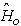is the "relevant" (or secular) part composed of the Hamiltonian for the free subsystems and a part of the interactions, namely, those strong enough to have associated very short relaxation times (meaning those much smaller than the characteristic time scale of the experiment (or resolution time), and possessing certain symmetry properties described below. The other term,¢, contains the interactions related to long-time relaxation mechanisms. The symmetry characteristics of the strong interactions depend on the problem under consideration: The required symmetry - to be called Zubarev-Peletminskii symmetry condition - is thatwhere the left side is the commutator ofand, and the ajk are c-numbers determined by. It should be noticed that quantitiescan be dependent on the space variable, i.e., when they are densities and then quantities a can also be differential operators.

Equation (2) provides a closure-like condition for the choice of the set of variables. In reference  this procedure is related to the question of the choice of the basic variables in phenomenological irreversible thermodynamics. Practical use of the formalism usually requires to introduce an appropriated truncation procedure (see second of references ) along the chain that application of the method produces, or an asymptotic expansion yielding renormalized coefficients. It ought to be noticed that Eq.(2) also encompasses the case of quantitiessuch that they have associated null coefficients a; i.e., they are constants of motion under the dynamics generated by. Accordingly they are acceptable basic variables, anditself falls under this condition, and should be always present in the basic set.

Assuming that the basic set {} has been chosen, the nonequilibrium statistical operator is built in the MaxEnt-NESOM, i.e. within the context of Jaynes' Predictive Statistical Mechanics, using the principle of maximization of the statistical-informational entropy, with fading memory and ad hoc hypothesis which introduce from the outset irreversible evolution from an initial condition of preparation of the system: for details see references [2, 3, 5, 6]. Summarizing for later use, in the particular case of Zubarev's approach to the MaxEnt-NESOM [2, 13] (by far the most concise and practical one, and founded on sound physical basis) the nonequilibrium statistical operator is given by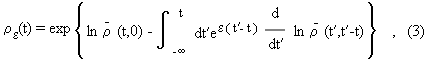whereis an auxiliary operator (or coarse-grained part of re(t)) - of large practical relevance in the theory - given by the Gibbsian-like generalized nonequilibrium canonical distribution

-
r (t,0) = exp ì
í
î - f(t) - å
j ó
õ d3r Fj(r,t) ^
P j (r) ü
ý
þ , (4a)

andIn Eq.(4), f(t) ensures the normalization of, and the Fj(t) (as well as f) are the Lagrange multipliers that MaxEnt introduces. In Eq.(3) e is a positive infinitesimal that goes to zero after the calculation of the averages is performed; this implies to introduce Bogoliubov's quasi-averages procedure , a symmetry-breaking process, in this case corresponding to a breaking of time-reversal symmetry in Liouville equation [2, 3, 5, 11]. Space dependence has been explicitly introduced, and in the Eq.(3) it is clear the presence of retro-effects with fading memory, where quantitiesare given in the time-dependent Heisenberg representation. Variables Fj(t) are related to the macrovariables Qj through the relations

Qj(r,t) = Tr ì
í
î ^
P j (r) re(t) ü
ý
þ , (5)

with j = 1, 2, ..., and the method allows for the construction of a quantum nonlinear, nonlocal in space, and memory dependent kinetic theory describing information on the dissipative evolution of the macroscopic nonequilibrium state of the system [2, 3, 13, 15].

Let us consider the construction of the MaxEnt-NESOM nonlinear quantum kinetic theory. First, it should be noticed that the equations of evolution for the basic variables are simply the time derivative of Eq.(5), namely,that is to say, they are the average over the nonequilibrium ensemble of the corresponding Heisenberg equation of motion for quantities(r). Equation (6), taking into account that j = 1, 2, ..., constitutes, in general, a coupled set of integro-differential equations of formidable proportions. But the use of the separation of the Hamiltonian as provided by Eq.(1), and the closure condition of Eq.(2), allows to obtain an alternative expression for the right hand side of Eq.(6) easier to handle mathematically and allowing to obtain a more clear physical picture of the dissipative processes that develop in the media. A price is paid, consisting in the fact that we obtain an infinite series of collision operators associated to two, three, etc, collisional processes, but at the same time permits evaluation of the different contributions and to introduce a cut-off in the series expansion. This, of course, amounts to an approximation and therefore each particular case requires an evaluation of its validity .

We briefly summarise the results: Taking into account that the Liouville equation with infinitesimal sources in Zubarev's approach to the MaxEnt-NESOM, is given by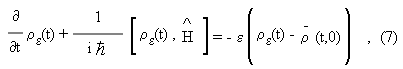where re is given in Eq.(3) andin Eq.(4), substracting from both sides the quantityand multiplying both sides of the resulting equation on the left by expand on the right by its adjoint, and then by eet, and resorting to Zubarev-Peletminskii symmetry condition of Eq.(2) together with some mathematical manipulations (Appendix A in ), we obtain thatwhere d stands for functional derivative and subindex nought indicates that the dynamical operators are given in interaction representation, that is, in Heisenberg representation with the partial Hamiltonian, and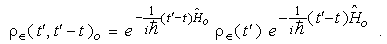Equation (9) is an integral equation for re(t) and admits a solution by iteration, namely,

re(t) = ¥
å
m = 0 re(m)(t,0) , (10)

as described in reference . Using the partial solutions re(m) of Eq.(10), as given in , in Eq.(6) leads to equations of evolution of the type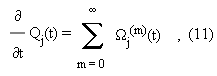where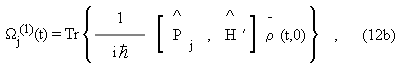are the first three contributions, which are those of interest in what follows, and then we omit to write down the other cumbersome higher order contributions. We stress that Eqs.(11), which are highly nonlinear, are applicable to a large array of situations in systems far-from-equilibrium conditions, contain nonlocal in space contributions (space correlations) and memory (time correlations), and can be considered as far-reaching generalizations of Mori's equations . It may be noticed that this is a kind of fading memory (as a result of the presence of the kernel in the form of the exponential), or, alternatively, can be interpreted as a time-smoothing procedure in Kirkwood's sense . Contributions W(0) and W(1) are - in Mori's terminology - precession terms, while W(2) is a proper collision integral, and the first one in Eq.(11) to contribute to dissipation (W(0) and W(1) are dissipationless contributions).

Again, because of the fundamental tenets of the method, mainly Eqs.(1) and (2), the collision integral of Eq.(12c), which, clearly, contains (fading) memory effects, can be rewritten in terms of only the auxiliary operator(t,0) at the time t when a measurement is performed. For that purpose we introduce what we call a memory-propagating operator Q in such a way that

-
r (t¢,t¢-t) = Q(t;t¢-t) -
r (t,0) , (13)

with the details of the calculation of this memory-propagating operator given in reference . We simply notice that it can be written in the form

Q(t;t) = 1 + ¥
å
k = 1 Q(k)(t;t) , (14)

where t º t¢-t and Q(k) is a correlation function in a k-power order in the interaction strengths in. The first term, namely the unit operator 1, does not propagates memory, the latter being accounted for in the series of Q(k) op1erators of k-power ( k = 1, 2,...) in the strengths of the interactions contained in. Therefore the memoryless limit corresponds to the one of weak coupling between subsystems.

Futhermore, as shown elsewhere , the collision integrals W(m) for m ³ 2, can be rewritten in terms of simpler collision integrals in the form

Wj(m)(t) = ¥
å
n = m (m)Jj(n)(t) . (15)

Without going into details (some considerations are given in  and further discussions will be reported in a forthcoming article), it is verified that the partial collision integrals J(n) are composed of three types of contributions, namely, (i) a main one corresponding to the Born series in perturbation theory for nth-order collisions averaged over the nonequilibrium ensemble; (ii) a second one which propagates the effect of the change in the nonequilibrium thermodynamic state of the system during its evolution while the mechanical collisions described by (i) are occurring; and (iii) corrections coming from memory effects, which, as noticed, since m ³ 2, are of order three and up in the interaction strengths. These three different contributions may be termed as dissipation effects arising out of: (i) the result of "viscous" forces; (ii) accounted for the change in the variables that characterize the evolving macrostate; and (iii) the effect of the evolving history (or memory) of a fading character implying in irreversible thermodynamic behavior of the system.

Let us concentrate our attention in the term with m = 2, and for simplicity let us retain only the contribution of type (i), in this case implying in the Golden Rule of Quantum Mechanics averaged over the nonequilibrium ensemble. In a large number of cases, as the one to be considered in next section, the contribution of type (ii) cancels out because of the properties of the subsystem that acts as an ideal reservoir. Finally, neglecting the contribution of type (iii) implies in a memoryless approximation: this means that in Eq.(14) we keep only the term 1 and, then, the contribution from this collision integral is exclusively of second order in the interaction. The resulting collision integral, to be designated by J(2) is [13, 15]Consequently the memoryless (Markovian) kinetic equation is, in MaxEnt-NESOM, and in the conditions stated above implying that J(1) = 0, given bywhere we wrote Jj(0)(t) instead of the Wj(0)(t) of Eq.(12a). Let us next apply these results to a particular system.

III A Spin-Lattice System

Let us consider a system of N spins in interaction with a lattice, the latter composed of a gas of phonons at temperature To and to be considered as an ideal reservoir, and in the presence of a magnetic field B = (Bx, By, Bz). Spin-lattice relaxation is of fundamental relevance in the area of eletronic paramagnetic resonance, the first studies dating back to the work of Waller in 1932. Two types of processes were proposed, a direct one with absorption or emission of a phonon, and a so-called Raman process with scattering of phonons. The theory was extended by Van Vleck on the basis of the study of the effect arising out of the modulation of the crystalline eletrostatic potential. We are considering here an ideal model involving only the direct process mentioned above . In this case the Hamiltonian is of the form of Eq.(1), where now 

^
H o = ^
H S + ^
H R (18)

with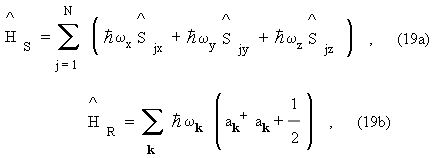and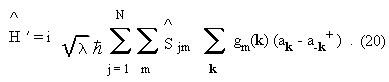In Eq.(20), m = x,y,z, in Eq.(19a) wx,y,z are the Larmor frequencies of the spins in the magnetic field, andS accounts for the precession of the spin around the magnetic field. Moreover,x,y,z are the spin-half operators, a (a+) boson annihilation (creation) operators; l is a coupling constant, gm(k) the matrix elements of the interaction between spin and thermal bath, and HR the Hamiltonian of the free phonons with frequency dispersion relation wk. The choice wy = 0, gx = gy = 0, correspons to the model Hamiltonian used in the theory of paraeletric resonance and relaxation, where them stands for isospin; see for example , and in what follows for simplicity we take an isotropic, gx = gy = gz = g, model.

The chosen set of basic dynamical variables for the spin system is composed of 3N spin operators, namely, {jx,jy,jz, j = 1, 2, ..., N }, we call ájm| t ñ the corresponding macrovariables, and Fjm(t) the associated Lagrange multipliers. The given basic dynamical variables satisfy Zubarev-Peletminskii closure condition of Eq.(2); in factfor each j = 1, 2, ..., N. For the thermal bath, the basic variable is the HamiltonianR and, since it is assumed to constantly remain in equilibrium, it is statistically characterized by a canonical distribution, in which, the associated Lagrange multiplier is bo = 1/kB To. Moreover, [R ,o ] = 0. On the other hand, the contribution to the spin dynamics due to the interaction isfor each j = 1, 2, ..., N. For such choice of basic variables the auxiliary MaxEnt-nonequilibrium statistical operator is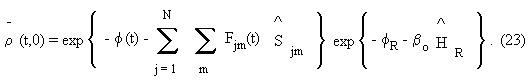This coarse-grained statistical operator is the direct product of the statistical operator for the spin system times the one for the thermal bath, the latter being the equilibrium canonical distribution as already noticed. We recall that f and fR ensure the normalization of each one respectively, and play the role of the logarithm of partition functions in this nonequilibrium ensemble formalism.

Applying the memoryless approximation of Eq.(17), once in this case it is in fact verified that all four contributions of the type J(1) [cf. Eq.(12b)] are null, it follows in a matrix form which is the same for each spin (j = 1, 2, ..., N), thatwhere M(t) is the column vector with components áx| t ñ, áy| t ñ and áy| t ñ; a is the nonhomogeneous term in this equation, namely, the column vector of components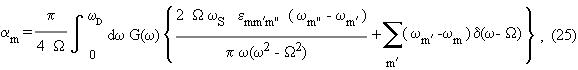where

ws = wx + wy + wz , (26a)

W2 = wx2 + wy2 + wz2 , (26b)

and

G(w) = l |g(w)|2 D(w) , (26c)

where D(w) is the density of phonon states, and g(w) the matrix element g(k) in frequency space in this isotropic model. In Eq.(25), m ¹ m¢ ¹ m", m, m¢, m" = x, y, z; emm¢m" = 1 if mm¢m" is a ciclic permutation of xyz (i.e., xyz, zxy, and yzx) and null otherwise, wD is Debye cut-off frequency. The square matrix A is

A = é
ê
ê
ê
ê
ë - gx - wz + ax wy + ax wz + ay - gy - wx + ay - wy + az wx + az - gz ù
ú
ú
ú
ú
û , (27)

where the quantities gm and am are given by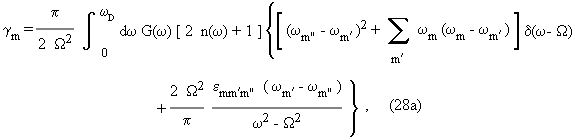Finally,is the population of the phonon modes (Planck distribution with temperature To).

Using the method of the matriçant , the solution of Eq.(24) is

M(t) = - A-1 ( 1 - exp{ t A}) a , (30)

once we take into account that the initial values are null, i.e. M(0) = 0. From this Eq.(30) we can easily see that a steady state solution can follow only if for sufficiently large t (i.e. for t much larger than all relaxation times)

exp{ t A } a ® 0 , (31)

and then a steady-state (ss) magnetization follows, given by

Mss = - A-1a , (32)

Consequently, the behavior of the trajectories [the evolution of M(t)] is dependent on the condition of Eq.(31), and this is governed by the eigenvalues of the matrix A (or Lyapounov exponents in linear stability analysis ). For Eq.(31) to follow all Lyapounov exponents must have a negative real part (its modulus standing for the reciprocal of a relaxation time), while no steady-state may occur if at least one of Lyapounov exponents has a positive real part (when the spins keep precessing indefinitely around the resulting magnetic field). Let us consider the matrix of Eq.(27), whose eigenvalues mi are, for W < wD, given by the expressionswhere

h = W2 - ( wxwy +wxwz + wywz ) ³ 0 , (34a)

(then m1< 0),

D = m12 - 4 ( W2 - 2 h k) , (34b)

andInspection of Eqs.(33) tells us that for a steady state to follow is required that either

D(l) = m12 + 8 h k-4 W2< 0 , (35a)

(an inequality satisfied, for all other parameter being fixed, for l < l+, where l+ is the largest value of the solutions of equation D(l) = 0), when, since m1< 0, the eigenvalues m2,3 have a rotating solution with frequency | D |1/2, and a decay time 2 / |m1|, or D(l) > 0, and

W2> 2 h k , (35b)

what means that m2,3 are real negative numbers.

Consider now the steady state, which is the final state of equilibrium with the lattice (see Appendix A). After some lengthly but straightforward algebra, we find that the components of the magnetization in the steady state are: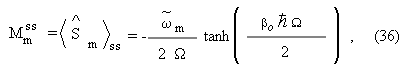where

~
w m = wmcm , (37)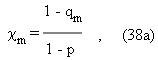To be consistent with the fact that the Markovian limit is valid only in first order in l (second order in the interaction strengths), we expand 1/(1 - p) in a series of powers of l around l = 0 and take only terms up to first order, i.e. 1/(1 - p) = 1 + p + O(l2). Consequently, up to first order in l, Eq.(38a) takes the form

cm = 1 + p - qm , (39)

and then the renormalized Larmor frequencies are

~
w m = wm + Dwm , (40)

where

Dwm = wm ( p - qm ) . (41)

Moreover, taking account Eqs.(36) and (40) we find thatas it should. This is so because we have neglected contributions of type O(l2) to be consistent with the Markovian approximation. The contribution linear in l (second order in the interaction strength), inx2 +y2 +z2, that is, wxDwx + wyDwy+ wzDwz, [cf. Eqs.(37) and (38)] cancels out, while those of order O(l2) do not. Overlooking this point led Luczka  to the wrong conclusion that the Markovian limit in Zubarev's NESOM has not been properly derived. He claims that Eq.(24) is incorrect, because from it there follow unphysical results, that is, the Lagrange parameters Fm (bn in his nomenclature) cannot in all circumstances be real numbers as they should. In other words, according to Luczka the Markovianization process in Zubarev's approach does not, in this case, satisfy that the sum of the squares of the steady state values of the spin variables is smaller or at most equal to one fourth. An alternative Markovianization procedure is attempted by Luczka resorting to a modified version of Davies' technique . Equations of the form of Eq.(24) are obtained but with a modified matrix A and vector a, which apparently lifts the above mentioned claimed incorrectness of the Markovianization procedure described in the previous section. However, the modified terms lead to new equations of evolution for the magnetization which are not Heisenberg equations of motion for the spins averaged over the nonequilibrium ensemble as it should, which are our Eqs.(24), but contain additional (spurious) terms. This evidently points to some mistake in Luczka's treatment of the problem, which, as noticed, resides in that a failure of consistency in the calculation has been introduced, consisting in the fact that he obtains the value of |Mss|2 larger than 1/4, but as a consequence of the presence of terms O(l2).

Let us now look into the relevant question of analyzing the Lagrange multipliers Fm(t). A straightforward calculation leads to the result thatwhere

F2(t) = Fx2(t) + Fy2(t) + Fz2(t) . (44)

Using Eqs(43) and (44), it follows that the Lagrange multipliers can be expressed in the formwhere M(t) is the solution of the equations of evolution for the magnetization, as given by Eq.(30), and |M(t)|2 = Mx2(t) + My2(t) + Mz2(t). In the steady state regime it follows, up to first order in l (second order in the interaction strengths), thatwherem are the renormalized Larmor frequencies given by Eqs.(40) and (41). We note that the Lagrange multipliers can be rewritten aswhere bm plays the role of a kind of inverse of temperature for the m-component of magnetization, which we write as

bm = bo + Dbm , (48)

whereand p and qm are given in Eqs(38b). and (38c).

Finally, we note that using Eq.(49) in Eq.(23) - the one that defines the auxiliary "coarse-grained" operator - and introducing the later in the expression that defines Zubarev's statistical operator in the steady state, ress, one obtains a "fine-grained" statistical operator which coincides with the canonical distribution in equilibrium at temperature To. This is to be interpreted in the sense that both produce the same average values, over the ensemble, of any observable, as shown in Appendix A.

IV Concluding Remarks

As stated in the Introduction, we have here reconsidered the question of the derivation of a generalized nonlinear kinetic theory based on the seemingly powerful, concise and practical, soundly based, MaxEnt-NESOM. We recall that the MaxEnt-NESOM is a formalism which can be considered to be encompassed within the scope of Jaynes'Predictive Statistical Mechanics, and which provides microscopic mechanical-statistical foundations to phenomenological nonequilibrium thermodynamics and hydrodynamics in the form of so-called Informational Statistical Thermodynamics and Non-Classical Thermo-Hydrodynamics, as well as a response function theory for systems far away from equilibrium, and which is showing to be of particular success for analyzing a large class of experimental situations. In all these situations the kinetic theory mentioned above - or, more precisely, the equations of evolution for the basic macrovariables that characterize the macrostate of the system - plays a fundamental role.

It has been previously noticed, and we further stress the point, that such MaxEnt-NESOM transport theory can be considered a far-reaching generalization of Boltzmann's approach  and Mori's formalism [12,15]. It can properly account for a large array of arbitrarily far-from-equilibrium situations involving nonlinearity, nonlocality-in-space (space correlations), and history (or memory or retro-) effects (time correlations), containing limiting cases (that is, restricted theories) within its scope as, for example, Boltzmann's original equation, Mori's equations, Maxwell-Cattaneo-Vernotte's equations, the equations of traditional Hydrodynamics, Fokker-Planck equations, several approaches to master equations, etc..

The theory has been very briefly reviewed in section II, followed by a description of a kinetic theory, with particular attention to the limit of taking in the derivation the lowest order in the interacting coupling constant between subsystems. As shown, this is the memoryless approximation in the theory (the sometimes called Markovian kinetic equations), which, we stress, are shown to be acceptable in the weak-coupling limit. They are very useful equations which apply to a large set of experimental situations, for example, the case of the highly excited photoinjected plasma in semiconductors (a system of large technological, industrial and economical interest besides the purely scientific one), as reviewed in reference .

We have shown that the MaxEnt-NESOM equations of evolution are composed, in the memoryless limit, of a contribution that can be interpreted as the Golden Rule of Quantum Mechanics (involving two-particle collisions) averaged over the nonequilibrium ensemble plus a contribution arising out of the change in time of the macroscopic variables that characterize the macroscopic state of the system. This last contribution has been omitted when writing Eq.(18), on the basis that in most cases of interest it vanishes as a result of symmetry considerations related to the description of the macrostate of the system. Moreover, contributions due to memory effects are of course absent in this memoryless approximation, but are present in the contributions of higher order than two in the interaction strengths. Contributions to the collision integrals arising out of memory effects are at least of third order (usually beginning with the fourth order) in the interaction strengths and then, as already noticed before, can be neglected in the weak coupling limit.

Finally, in section III we have applied the theory to a specific model for a spin system in interaction with a thermal reservoir composed of the lattice vibrations in the material. The equations of evolution for the variables corresponding to the average of the spin dynamical variables are derived in the memoryless limit. They are exactly solved and it is shown that depending on the characteristics of the material it may follow either a steady state or a persistent precessional motion. This depends on the fact that the constant rate of energy pumped on the system (via the coupling with the external field) may or may not, respectively, be dissipated towards the reservoir. The complete solution for the evolution of the magnetization is obtained, as well a the correct final state of equilibrium of the spin system at the lattice at temperature To.

In the process, we have compared the results we have obtained, for the system of section III, with those of Luczka . This author mantained that the Markovian approach as derived by Zubarev, Peletminskii, and us is incorrect. As discussed in the previous section such consideration is invalid, and the result of a failure of consistency in the order of the approximations introduced. In a forthcoming article we compare the MaxEnt-NESOM and Davies  treatment of the Markovian limit, showing their equivalence in the weak-coupling limit.

Acknowledgments

We acknowledge finantial support to our Group that in different opportunities was provided by the State of São Paulo Research Foundation (FAPESP); the National Research Council (CNPq); Unicamp Foundation (FAEP); the Ministry of Planning (FINEP); IBM-Brasil; the John Simon Guggenheim Memorial Foundation (New York, USA). One of the authors (JRM) is a FAPESP Post-Doctoral Fellow, and other two (ARV and RL) are CNPq Research Fellows.

The "fine-grained" Zubarev's statistical operator in the final steady state is

where

^
G o = bo ^
H R + bo ^
H S +bo å
j,m (h/2p) Dwm ^
S jm , (A.2)

andwitho =R +S. Noticing thatthenUsing Eq.(A.5) in Eq.(A.3), and integrating by parts, it follows that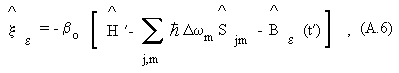where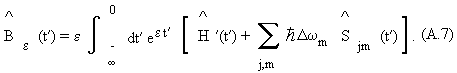Hence, the statistical operator in the steady state, as given by Eq(A.1) becomesan then the average of any observablein this steady state is given byThis separation is obtained applying Heims-Jaynes expansion series for averages , where the first term on the right is the average over the canonical ensemble, with

Z = Tr {exp( - bo) } being the canonical partition function. The second term, involving the e® +0 limit, contains the terms of the series in Heims-Jaynes expansion involving operator Be once, twice, etc. Let us consider the contribution of this operator in the first term, namely,where áñc is the average over the canonical ensemble.

We consider first the contribution to Be in Eq.(A.7), and present in Eq.(A.10), containing¢(t¢). According to Abel's theorem

lim
t¢® - ¥ m | ^
H ¢(t¢) | m¢ = lim
e® +0 e ó
õ 0

- ¥ dt¢ ee t¢ m | ^
H ¢(t¢) | m¢ (A.11)

for any matrix element in, say, states | m ñ. But this limit is zero because of, in Zubarev's MaxEnt-NESOM approach, the boundary condition

lim
t¢® - ¥ ì
í
î lnre(t + t¢,t¢) -ln -
r (t + t¢,t¢) ü
ý
þ = 0 , (A.12)

in the initial value problem including Bogoliubov's principle of correlation weakening , or, alternatively, taking adiabatic switching on of the interaction at to® -¥.

For the other contribution containing the spin operators in Eq.(A.10), a calculation performed taking the axis of spin quantization along the axis with directional cosines

zx = wx / W, zy = wy / W, and zz = wz / W, lead to the result that it is proportional to wxDwx +wyDwy + wzDwz, which is zero [see main text after Eq.(42)], and finally in Eq.(A.9)Therefore, the average value of any observablewith eitheror the canonical distribution coincide, thus defining the same macroscopic state. Hence, the steady state is the state of equilibrium with the lattice.

References


• R. Zwanzig, Where do we go from here ? in Perspectives in Statistical Physics, edited by H. J. Ravechè (North Holland, Amsterdam, 1981).
• 
• D. N. Zubarev, V. N. Morosov, and G. Röpke, Statistical Mechanics of Nonequilibrium Phenomena, Vol.1: Basic Concepts, Kinetic Theory (Akademie Verlag, Berlin, 1996).
• 
• R. Luzzi and A. R. Vasconcellos, On the Nonequilibrium Statistical Operator Method, Fortschr. Phys. / Prog. Phys. 38, 887 (1990).
• 
• E. T. Jaynes, Predictive Statistical Mechanics, in Frontiers of Nonequilibrium Statistical Physics, edited by G. T. Moore and M. O. Scully (Plenum, New York, 1986); Macroscopic Predictions, in Complex Systems: Operational Approaches, edited by H. Haken (Springer, Berlin, 1985).
• 
• J. T. Alvarez-Romero and L. S. Garcia-Colin, The Foundation of Informational Statistical Thermodynamics Revisited, Physica A 232, 207 (1996).
• 
• R. Luzzi, A. R. Vasconcellos, and J. G. Ramos, Considerations on a Nonequilibrium Ensemble Formalism, IFGW-Unicamp Internal Report (1997), and future publication.
• 
• L. S. Garcia-Colin, A. R. Vasconcellos, and R. Luzzi, On Informational Statistical Thermodynamics, J. Non-Equilib. Thermodyn. 19, 24 (1994).
• 
• S. Sieniutycz and P. Salamon, Editors, Introduction and following articles in Nonequilibrium Theory and Extremum Principles (Taylor and Francis, New York, 1990); also, R. E. Nettleton and S. L. Sobolev, Application of Extended Thermodynamics to Chemical, Rheological, and Transport Processes, J. Non-Equilib. Thermodyn. 20, 205 (1995).
• 
• A. R. Vasconcellos, R. Luzzi, and L. S. Garcia-Colin, A Microscopic Approach to Irreversible Thermodynamics I, Phys. Rev. E 43, 6022 (1991); II, ibid E 43, 6633 (1991); R. Luzzi and A. R. Vasconcellos, The Basic Principles of Irreversible Thermodynamics in an Informational Statistical Approach, Physica A 241, 677 (1997); M. A. Tenan, A. R. Vasconcellos and R. Luzzi, Statistical Mechanical Foundations for a Generalized Thermodynamics of Dissipative Processes, Fortschr.Phys./Prog. Phys. 45, 1 (1997); R. Luzzi, A. R. Vasconcellos and J. G. Ramos, Statistical Foundations of Irreversible Thermodynamics, Fortschr. Phys./Prog. Phys. in press.
• 
• V. P. Kalashnikov, Teor. Mat. Fiz. 9, 94 (1971) [Theor. Math. Phys. 9, 1003 (1971)].
• 
• J. G. Ramos, A. R. Vasconcellos, and R. Luzzi, A Classical Approach in Predictive Statistical Mechanics: A Generalized Boltzmann Formalism, Fortschr. Phys. / Prog. Phys. 43, 265 (1995).
• 
• J. R. Madureira, A. R. Vasconcellos, R. Luzzi, J. Casas-Vázquez, and D. Jou, Evolution of Nonequilibrium Processes in a Statistical Thermodynamic Approach, IFGW-Unicamp Internal Report (1997) and J. Chem. Phys. 108, 7568 (1998); ibid 108, 7580 (1998).
• 
• D. N. Zubarev, Nonequilibrium Statistical Thermodynamics (Consultants Bureau, New York, 1974).
• 
• A. I. Akhiezer and S. V. Peletminskii, Methods of Statistical Physics (Pergamon, Oxford, 1981).
• 
• L. Lauck, A. R. Vasconcellos and R. Luzzi, A Nonlinear Quantum Transport Theory, Physica A168, 789 (1990).
• 
• In Memory of Dmitrii Nikolaevich Zubarev (November 27, 1917 - July 29, 1992), Editorial Preface in Teor. Mat. Fiz. 96, 321 (1993) [Theor. Math. Phys. 96, 995 (1994)].
• 
• A. C. Algarte, A. R. Vasconcellos, and R. Luzzi, Kinetics of Hot Elementary Excitations in Photoexcited Polar Semiconductors, Phys. Stat. Solidi (b)173, 487 (1992); Ultrafast Phenomena in the Photoinjected Plasma in Semiconductors, Braz. J. Phys. 26, 543 (1996).
• 
• J. Luczka, On Markovian Kinetic Equations: Zubarev's Nonequilibrium Statistical Operator Approach, Physica A149, 245 (1988).
• 
• N. N. Bogoliubov, Problems of a Dynamical Theory in Statistical Physics, in Studies in Statistical Mechanics I, edited by J. de Boer and G. E. Uhlenbeck (Noth Holland, Amsterdam, 1962); for additional discussions and applications see: G. E. Uhlenbeck, in Lectures in Statistical Mechanics, edited by M. Kac (Am. Math. Soc., Providence, RI, 1963); L. L. Buishvili and M. D. Sviadaze, On the Quasi-Thermodynamic Theory of Magnetic Relaxation, Physica 59, 697 (1972); A. R. Vasconcellos, A. C. Algarte, and R. Luzzi, On the Relaxation-times Hierarchy in Dissipative Systems: An Example from Semiconductor Physics, Physica A166, 517 (1990).
• 
• H. Mori, Transport, Collective Motion, and Brownian Motion, Prog. Theor. Phys. (Japan) 33, 423 (1965).
• 
• N. N. Bogoliubov, Lectures on Quantum Statistical (Gordon and Breach, New York, 1970)
• 
• J. G. Ramos, A. R. Vasconcellos, and R. Luzzi, On the Truncation Procedure in the Nonequilibrium Statistical Operator Method, IFGW-Unicamp Internal Report (1998), and future publication.
• 
• J. G. Kirkwood, The Statistical Mechanical Theory of Transport Processes, J. Chem. Phys. 14, 180 (1946); ibid. 15, 72 (1946).
• 
• I. Waller, Z. Phys. 79, 730 (1932); J. H. Vleck, Phys. Rev. 57, 426 (1940); R. Orbach and H. J. Stapleton, Electron Spin-Lattice Relaxation, in Electron Paramagnetic Resonance, edited by S. Geschwind (Plenum, New York, 1972).
• 
• L. M. Sander and H. B. Shore, Theory of Paraelectric Resonance and Relaxation, Phys. Rev. B 3, 1472 (1971).
• 
• F. R. Gantmacher, The Theory of Matrices, Vol.2, Ch. XIV, Sect. 5 (Chelsea, New York, 1974).
• 
• W. E. Boyce and R. C. DiPrima, Elementary Differential Equations and Boundary Value Problems (Wiley, New York, 1992).
• 
• E. B. Davies, Markovian Master Equations, Commun. Math. Phys. 39, 91 (1974).
• 
• S. P. Heims and E. T. Jaynes, Rev. Mod. Phys. 34, 143 (1962), see pp. 148-150 and Appendix B.
• 
• D. N. Zubarev, M. V. Morosov, and G. Röpke, in reference , Sections 2.3.6 and 2.4.3.

# Appendix A:   The Limit of Equilibrium• *

# Publication Dates

• Publication in this collection
13 May 1999
• Date of issue
Sept 1998